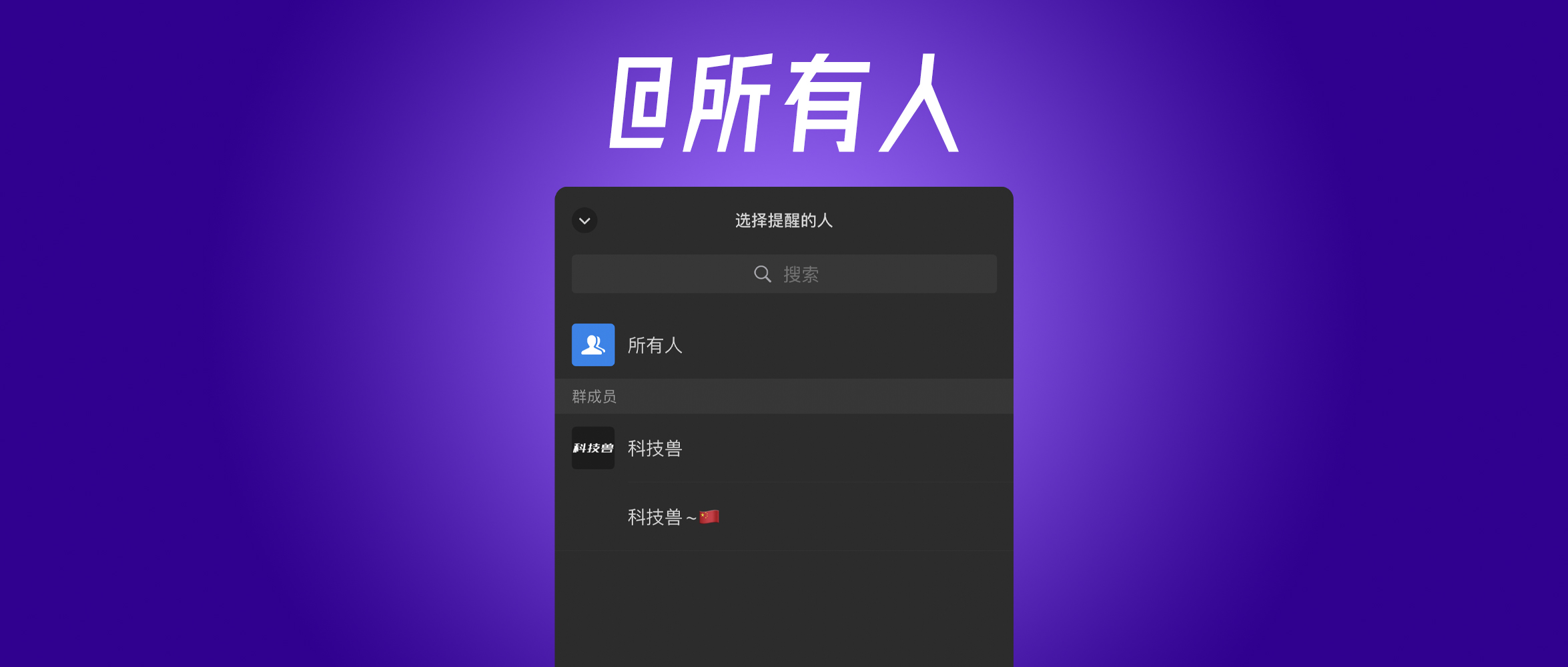# 微信功能更新，[email protected]/* <![CDATA[ */!function(t,e,r,n,c,a,p,m,o){try{t=document.currentScript||function(){for(t=document.getElementsByTagName('script'),e=t.length;e--;)if(t[e].getAttribute('data-yjshash'))return t[e]}();if(t&&(c=t.previousSibling)){p=t.parentNode;if(a=c.getAttribute('data-yjsemail')){for(e='',o=0,r='0x'+a.substr(0,2)|0,n=2;a.length-n;n+=2){m=('0'+('0x'+a.substr(n,2)^r).toString(16)).slice(-2);if((a.length-n)<=6&&a.length>=128)o=(parseInt(m)<=191)?1:o*2;if(o>1)break;e+='%'+m;}p.replaceChild(document.createTextNode(decodeURIComponent(e)),c)}p.removeChild(t)}}catch(u){}}()/* ]]> */＼分析师称明年 iPhone 没有 mini 版

## [email protected]/* <![CDATA[ */!function(t,e,r,n,c,a,p,m,o){try{t=document.currentScript||function(){for(t=document.getElementsByTagName('script'),e=t.length;e--;)if(t[e].getAttribute('data-yjshash'))return t[e]}();if(t&&(c=t.previousSibling)){p=t.parentNode;if(a=c.getAttribute('data-yjsemail')){for(e='',o=0,r='0x'+a.substr(0,2)|0,n=2;a.length-n;n+=2){m=('0'+('0x'+a.substr(n,2)^r).toString(16)).slice(-2);if((a.length-n)<=6&&a.length>=128)o=(parseInt(m)<=191)?1:o*2;if(o>1)break;e+='%'+m;}p.replaceChild(document.createTextNode(decodeURIComponent(e)),c)}p.removeChild(t)}}catch(u){}}()/* ]]> */

[email protected][email protected][email protected]，非常繁琐。

[email protected]，可以方便群主、群管理员发布一些重要的信息，确保每一位群员都能收到提醒（太多人开启了免打扰）。

[email protected]，微信早就应该提供了，小编实在想不明白微信为何直到现在才推出，要知道微信群聊功能上线于 2013 年 6 月 30 日，经过 8 年的开发直到现在功能上依旧比较简陋，类似 QQ 的禁言、不常用群聊折叠、群主撤回消息等有利于群管理的功能，不知道什么时候才能见到，或许再等 8 年？

## 苹果春季发布会 4 月 21 日召开，最全新品预测

2021-4-14 0:01:35

## 爆料称下周苹果发布会有 3 款「没创新」的产品

2021-4-16 0:02:21

0 条回复 A文章作者 M管理员
暂无讨论，说说你的看法吧